§ 10         Helicoid

[ Equation of a general helical surface ]        The parametric equation of the known space curve is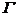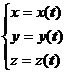The surface formed by the helical motion around the z -axis is called a general helical surface, and its equation is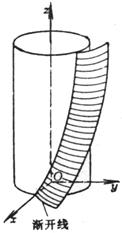Figure 7.21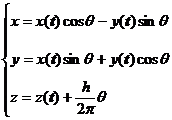where h is the pitch (or lead) .

[ Involute helical surface ] A surface formed by a known involute spiraling around the         z -axis on the Oxy plane is called an involute helical surface (Figure 7.21 ), and its equation is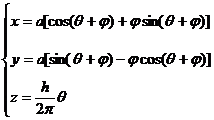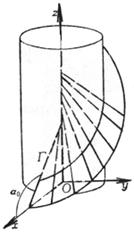Figure 7.22

where and are two parameters . " = constant" means different helixes on the surface, they have the same pitch h , but different helix angles; " = constant" means involutes at different positions .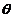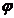[ Archimedes helical plane ]     A helical plane formed by a helical motion of a straight line intersecting the z -axis and at an angle of intersection with the Oxy plane is called an Archimedes helical plane (Figure 7.22 ), and its equation is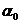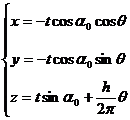Its intersection with the Oxy plane is an Archimedes spiral .